# 1.3 Hyperbolic functions

 Page 1 / 1

## Functions of the form $y=\frac{a}{x}+q$

Functions of the form $y=\frac{a}{x}+q$ are known as hyperbolic functions. The general form of the graph of this function is shown in [link] .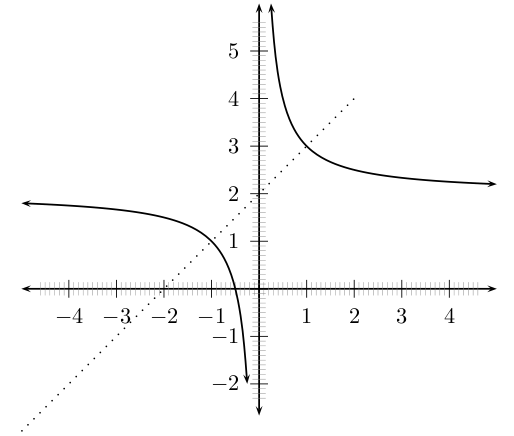General shape and position of the graph of a function of the form f ( x ) = a x + q .

## Investigation : functions of the form $y=\frac{a}{x}+q$

1. On the same set of axes, plot the following graphs:
1. $a\left(x\right)=\frac{-2}{x}+1$
2. $b\left(x\right)=\frac{-1}{x}+1$
3. $c\left(x\right)=\frac{0}{x}+1$
4. $d\left(x\right)=\frac{+1}{x}+1$
5. $e\left(x\right)=\frac{+2}{x}+1$
Use your results to deduce the effect of $a$ .
2. On the same set of axes, plot the following graphs:
1. $f\left(x\right)=\frac{1}{x}-2$
2. $g\left(x\right)=\frac{1}{x}-1$
3. $h\left(x\right)=\frac{1}{x}+0$
4. $j\left(x\right)=\frac{1}{x}+1$
5. $k\left(x\right)=\frac{1}{x}+2$
Use your results to deduce the effect of $q$ .

You should have found that the value of $a$ affects whether the graph is located in the first and third quadrants of Cartesian plane.

You should have also found that the value of $q$ affects whether the graph lies above the $x$ -axis ( $q>0$ ) or below the $x$ -axis ( $q<0$ ).

These different properties are summarised in [link] . The axes of symmetry for each graph are shown as a dashed line.

 $a>0$ $a<0$ $q>0$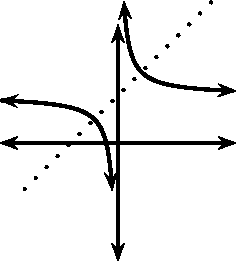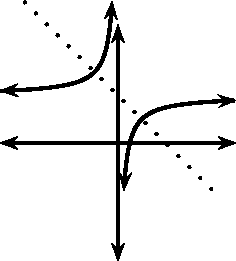$q<0$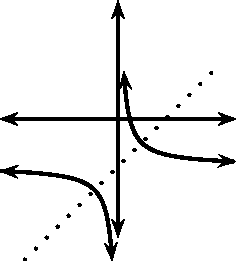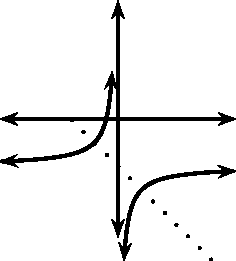## Domain and range

For $y=\frac{a}{x}+q$ , the function is undefined for $x=0$ . The domain is therefore $\left\{x:x\in \mathbb{R},x\ne 0\right\}$ .

We see that $y=\frac{a}{x}+q$ can be re-written as:

$\begin{array}{ccc}\hfill y& =& \frac{a}{x}+q\hfill \\ \hfill y-q& =& \frac{a}{x}\hfill \\ \hfill \mathrm{If}\phantom{\rule{3pt}{0ex}}x\ne 0\phantom{\rule{3pt}{0ex}}\mathrm{then}:\phantom{\rule{1.em}{0ex}}\left(y-q\right)x& =& a\hfill \\ \hfill x& =& \frac{a}{y-q}\hfill \end{array}$

This shows that the function is undefined at $y=q$ . Therefore the range of $f\left(x\right)=\frac{a}{x}+q$ is $\left\{f\left(x\right):f\left(x\right)\in \left(-\infty ;q\right)\cup \left(q;\infty \right)\right\}$ .

For example, the domain of $g\left(x\right)=\frac{2}{x}+2$ is $\left\{x:x\in \mathbb{R},x\ne 0\right\}$ because $g\left(x\right)$ is undefined at $x=0$ .

$\begin{array}{ccc}\hfill y& =& \frac{2}{x}+2\hfill \\ \hfill \left(y-2\right)& =& \frac{2}{x}\hfill \\ \hfill \mathrm{If}\phantom{\rule{4pt}{0ex}}x\ne 0\phantom{\rule{4pt}{0ex}}\mathrm{then}:\phantom{\rule{1.em}{0ex}}x\left(y-2\right)& =& 2\hfill \\ \hfill x& =& \frac{2}{y-2}\hfill \end{array}$

We see that $g\left(x\right)$ is undefined at $y=2$ . Therefore the range is $\left\{g\left(x\right):g\left(x\right)\in \left(-\infty ;2\right)\cup \left(2;\infty \right)\right\}$ .

## Intercepts

For functions of the form, $y=\frac{a}{x}+q$ , the intercepts with the $x$ and $y$ axis is calculated by setting $x=0$ for the $y$ -intercept and by setting $y=0$ for the $x$ -intercept.

The $y$ -intercept is calculated as follows:

$\begin{array}{ccc}\hfill y& =& \frac{a}{x}+q\hfill \\ \hfill {y}_{int}& =& \frac{a}{0}+q\hfill \end{array}$

which is undefined because we are dividing by 0. Therefore there is no $y$ -intercept.

For example, the $y$ -intercept of $g\left(x\right)=\frac{2}{x}+2$ is given by setting $x=0$ to get:

$\begin{array}{ccc}\hfill y& =& \frac{2}{x}+2\hfill \\ \hfill {y}_{int}& =& \frac{2}{0}+2\hfill \end{array}$

which is undefined.

The $x$ -intercepts are calculated by setting $y=0$ as follows:

$\begin{array}{ccc}\hfill y& =& \frac{a}{x}+q\hfill \\ \hfill 0& =& \frac{a}{{x}_{int}}+q\hfill \\ \hfill \frac{a}{{x}_{int}}& =& -q\hfill \\ \hfill a& =& -q\left({x}_{int}\right)\hfill \\ \hfill {x}_{int}& =& \frac{a}{-q}\hfill \end{array}$

For example, the $x$ -intercept of $g\left(x\right)=\frac{2}{x}+2$ is given by setting $x=0$ to get:

$\begin{array}{ccc}\hfill y& =& \frac{2}{x}+2\hfill \\ \hfill 0& =& \frac{2}{{x}_{int}}+2\hfill \\ \hfill -2& =& \frac{2}{{x}_{int}}\hfill \\ \hfill -2\left({x}_{int}\right)& =& 2\hfill \\ \hfill {x}_{int}& =& \frac{2}{-2}\hfill \\ \hfill {x}_{int}& =& -1\hfill \end{array}$

## Asymptotes

There are two asymptotes for functions of the form $y=\frac{a}{x}+q$ . Just a reminder, an asymptote is a straight or curved line, which the graph of a function will approach, but never touch. They are determined by examining the domain and range.

We saw that the function was undefined at $x=0$ and for $y=q$ . Therefore the asymptotes are $x=0$ and $y=q$ .

For example, the domain of $g\left(x\right)=\frac{2}{x}+2$ is $\left\{x:x\in \mathbb{R},x\ne 0\right\}$ because $g\left(x\right)$ is undefined at $x=0$ . We also see that $g\left(x\right)$ is undefined at $y=2$ . Therefore the range is $\left\{g\left(x\right):g\left(x\right)\in \left(-\infty ;2\right)\cup \left(2;\infty \right)\right\}$ .

From this we deduce that the asymptotes are at $x=0$ and $y=2$ .

## Sketching graphs of the form $f\left(x\right)=\frac{a}{x}+q$

In order to sketch graphs of functions of the form, $f\left(x\right)=\frac{a}{x}+q$ , we need to determine four characteristics:

1. domain and range
2. asymptotes
3. $y$ -intercept
4. $x$ -intercept

For example, sketch the graph of $g\left(x\right)=\frac{2}{x}+2$ . Mark the intercepts and asymptotes.

We have determined the domain to be $\left\{x:x\in \mathbb{R},x\ne 0\right\}$ and the range to be $\left\{g\left(x\right):g\left(x\right)\in \left(-\infty ;2\right)\cup \left(2;\infty \right)\right\}$ . Therefore the asymptotes are at $x=0$ and $y=2$ .

There is no $y$ -intercept and the $x$ -intercept is ${x}_{int}=-1$ .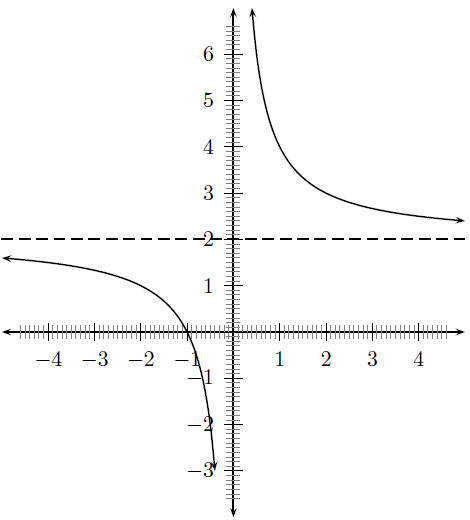Graph of g ( x ) = 2 x + 2 .

Draw the graph of $y=\frac{-4}{x}+7$ .

1. The domain is: $\left\{x:x\in \mathbb{R},x\ne 0\right\}$ and the range is: $\left\{f\left(x\right):f\left(x\right)\in \left(-\infty ;7\right)\cup \left(7;\infty \right)\right\}$ .
2. We look at the domain and range to determine where the asymptotes lie. From the domain we see that the function is undefined when $x=0$ , so there is one asymptote at $x=0$ . The other asymptote is found from the range. The function is undefined at $y=q$ and so the second asymptote is at $y=7$
3. There is no y-intercept for graphs of this form.
4. The x-intercept occurs when $y=0$ . Calculating the x-intercept gives:
$\begin{array}{ccc}\hfill y& =& \frac{-4}{x}+7\hfill \\ \hfill 0& =& \frac{-4}{x}+7\hfill \\ \hfill -7& =& \frac{-4}{x}\hfill \\ \hfill {x}_{\mathrm{int}}& =& \frac{4}{7}\hfill \end{array}$
So there is one x-intercept at $\left(\frac{4}{7},0\right)$ .
5. Putting all this together gives us the following graph:

## Graphs

1. Using graph (grid) paper, draw the graph of $xy=-6$ .
1. Does the point (-2; 3) lie on the graph ? Give a reason for your answer.
2. Why is the point (-2; -3) not on the graph ?
3. If the $x$ -value of a point on the drawn graph is 0,25, what is the corresponding $y$ -value ?
4. What happens to the $y$ -values as the $x$ -values become very large ?
5. With the line $y=-x$ as line of symmetry, what is the point symmetrical to (-2; 3) ?
2. Draw the graph of $xy=8$ .
1. How would the graph $y=\frac{8}{3}+3$ compare with that of $xy=8$ ? Explain your answer fully.
2. Draw the graph of $y=\frac{8}{3}+3$ on the same set of axes.

how do I set up the problem?
what is a solution set?
Harshika
find the subring of gaussian integers?
Rofiqul
hello, I am happy to help!
Abdullahi
hi mam
Mark
find the value of 2x=32
divide by 2 on each side of the equal sign to solve for x
corri
X=16
Michael
Want to review on complex number 1.What are complex number 2.How to solve complex number problems.
Beyan
yes i wantt to review
Mark
use the y -intercept and slope to sketch the graph of the equation y=6x
how do we prove the quadratic formular
Darius
hello, if you have a question about Algebra 2. I may be able to help. I am an Algebra 2 Teacher
thank you help me with how to prove the quadratic equation
Seidu
may God blessed u for that. Please I want u to help me in sets.
Opoku
what is math number
4
Trista
x-2y+3z=-3 2x-y+z=7 -x+3y-z=6
can you teacch how to solve that🙏
Mark
Solve for the first variable in one of the equations, then substitute the result into the other equation. Point For: (6111,4111,−411)(6111,4111,-411) Equation Form: x=6111,y=4111,z=−411x=6111,y=4111,z=-411
Brenna
(61/11,41/11,−4/11)
Brenna
x=61/11 y=41/11 z=−4/11 x=61/11 y=41/11 z=-4/11
Brenna
Need help solving this problem (2/7)^-2
x+2y-z=7
Sidiki
what is the coefficient of -4×
-1
Shedrak
the operation * is x * y =x + y/ 1+(x × y) show if the operation is commutative if x × y is not equal to -1
An investment account was opened with an initial deposit of $9,600 and earns 7.4% interest, compounded continuously. How much will the account be worth after 15 years? Kala Reply lim x to infinity e^1-e^-1/log(1+x) given eccentricity and a point find the equiation Moses Reply A soccer field is a rectangle 130 meters wide and 110 meters long. The coach asks players to run from one corner to the other corner diagonally across. What is that distance, to the nearest tenths place. Kimberly Reply Jeannette has$5 and \$10 bills in her wallet. The number of fives is three more than six times the number of tens. Let t represent the number of tens. Write an expression for the number of fives.
What is the expressiin for seven less than four times the number of nickels
How do i figure this problem out.
how do you translate this in Algebraic Expressions
why surface tension is zero at critical temperature
Shanjida
I think if critical temperature denote high temperature then a liquid stats boils that time the water stats to evaporate so some moles of h2o to up and due to high temp the bonding break they have low density so it can be a reason
s.
Need to simplify the expresin. 3/7 (x+y)-1/7 (x-1)=
. After 3 months on a diet, Lisa had lost 12% of her original weight. She lost 21 pounds. What was Lisa's original weight?
Got questions? Join the online conversation and get instant answers!By Sandhills MLTBy Sarah WarrenBy Janet ForresterBy Edgar DelgadoBy Saylor FoundationBy Yacoub JayoghliBy OpenStaxBy Lakeima RobertsBy Janet ForresterBy OpenStax